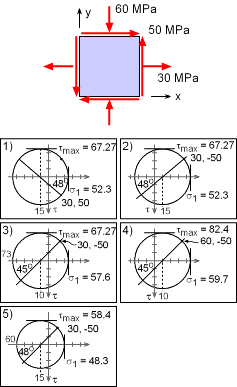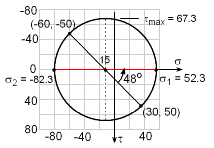Ch 7. Stress Analysis Multimedia Engineering Mechanics PlaneStress PrincipalStresses Mohr's Circlefor Stress Failure PressureVessels
 Chapter 1. Stress/Strain 2. Torsion 3. Beam Shr/Moment 4. Beam Stresses 5. Beam Deflections 6. Beam-Advanced 7. Stress Analysis 8. Strain Analysis 9. Columns Appendix Basic Math Units Basic Equations Sections Material Properties Structural Shapes Beam Equations Search eBooks Dynamics Fluids Math Mechanics Statics Thermodynamics Author(s): Kurt Gramoll ©Kurt GramollMECHANICS - EXAMPLEMohr's Circle Example Which Mohr circle is correct for the given stress state Solution The given stress components for the stress element are,        σx = 30 MPa      σy = -60 MPa      τxy = 50 MPaRotated Stress Element Mohr's Circle center will be at the normal stress average,      σaverage = [30 + (-60)]/2 = -15 MPa which is plotted on the diagram at the left. Next, a point on the circle is needed. Two different points can be used,      Point 1: (σx , τxy) = (30, 50)      Point 2: (σy, -τxy) = (-60, -50) Remember, the shear stress is plotted positive in the downward direction. From the plotted center and points on the circle, the radius (shear maximum) can be determined.      R = τmax = [(30+15)2 + 502]1/2 = 67.3 MPa Principal stresses are      σ1 = -15 + 67.3 = 52.3 MPa      σ2 = -15 - 67.3 = -82.3 MPa The principle direction is       tan 2θ1 = 50/(15+30)       2θ1 = 48.0o The correct diagram is 1)

Practice Homework and Test problems now available in the 'Eng Mechanics' mobile app
Includes over 400 problems with complete detailed solutions.
Available now at the Google Play Store and Apple App Store.